Math Concepts

# Complete Guide: Abacus Level 2

1.2k views

 1 Introduction 2 A brief history of Abacus 3 Abacus Counting & Techniques 4 Summary 5 Frequently Asked Questions (FAQs) 6 External References

13 November 2020

## Introduction

Necessity, it is said, is the mother of invention. And so came about the idea of the Abacus. The exact origin of the tool is not clear. It was important for traders and merchants to be able to count accurately, and counting boards were used to do so.

It is believed that the Counting boards are the predecessor of the beaded abacus. Counting boards were likely crudely constructed wooden tables, now lost to decomposition.

The Abacus is an ancient, simple calculating/computing tool still used all over the world. It's a very useful learning device for those who wish to learn the basics of arithmetic. It is also useful for the visually challenged. Mastering the abacus helps in performing addition, subtraction, multiplication, and division quickly.

The abacus comes in two forms, the first featuring one bead per column in the top row and four beads per column in the bottom, while the second features two beads per column on top and five beads per column on bottom.

## A brief history of Abacus

The oldest existing counting board was discovered on Salamis (Greece) on the island about a century ago. The original Greek abacus was a table with a raised edge, filled with sand.

It is thought that the word "abacus" comes from the Greek word "abax" meaning tray/table/tablet, which originated from the Semitic word "abq" which translates as dust/sand.

As time passed and cross-cultural exchanges took place, the abacus evolved as per the user’s requirements. The framework varied from country to country.

It is commonly believed that the Roman abacus was introduced into China by trading merchants. The earliest mention of the abacus in Chinese literature did not occur until the 12th century. In the middle ages counting tables was very common in Europe.

The Chinese abacus, or suan pan, is generally regarded as the earliest abacus with beads on rods. The Mandarin term Suan pan means calculating plate. The Suanpan is widely used throughout China and other parts of Asia.

The Suanpan features five-unit beads on each lower rod and two ‘five-beads’ on each upper rod.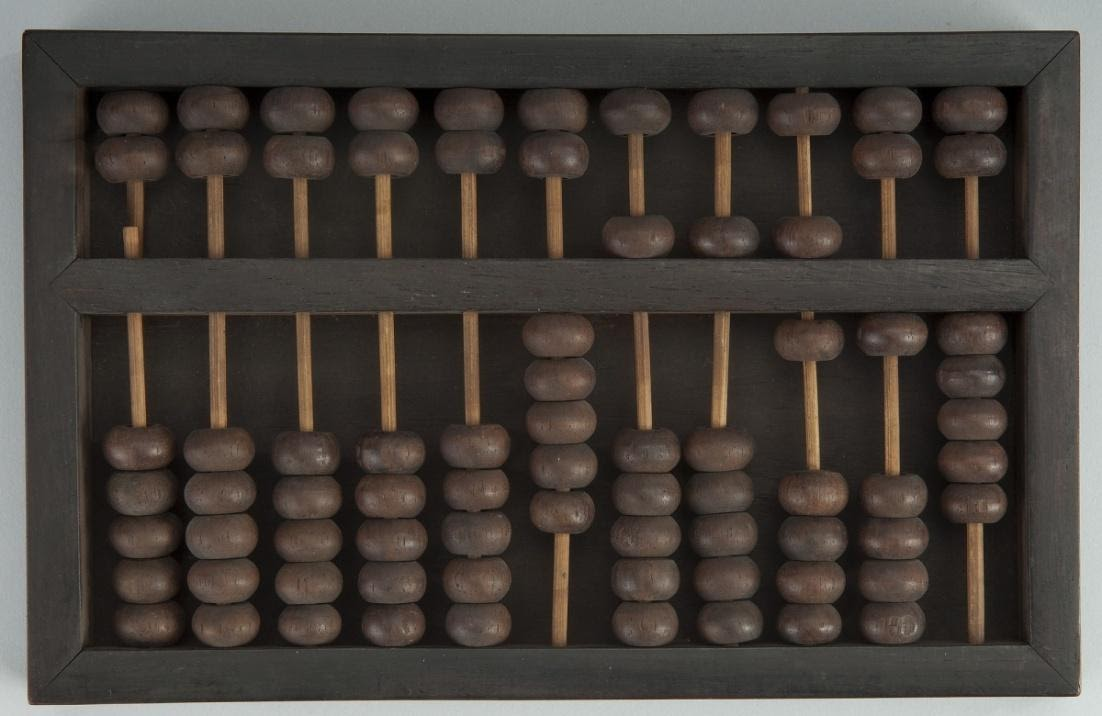The modern Japanese abacus, known as a Soroban, was developed from the Chinese Suanpan. The Soroban features four unit beads on the lower rods and one ‘five bead’ on the upper rods. The Soroban also features large sharp-edged beads that are easily manipulated, and the distance through which they move is relatively short, allowing for high-speed operation.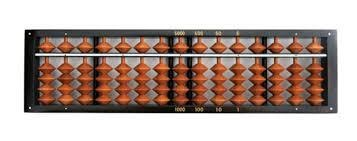The Russian abacus, the Schoty, features ten beads per rod and no dividing bar,. This requires the movement of a larger number of beads over a relatively far distance but is said to be easier to learn than instruments utilizing markers of value five. While all the previous devices were operated horizontally, the Russian instrument can also be operated vertically.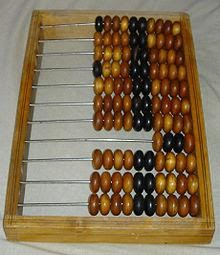Abaci are very much in use today. They have been invaluable in teaching number placement value. Not only that, but it also helps students have a better understanding of numbers.

Since the 1980s, the Chinese have begun to use the Soroban, the Japanese abacus. This is because the Soroban displays numbers in base 10 in a way that makes calculating easy. The ancient Chinese abacus is not as suitable for mathematical calculations as the Soroban.

## Abacus Counting & Techniques

In general, all abaci work in a similar fashion.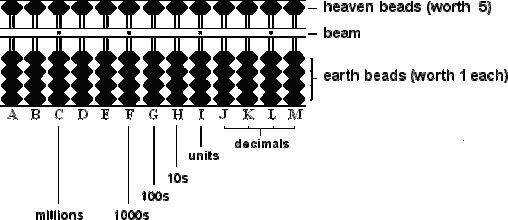In order to count on the abacus, slide all beads to the outer edge of their columns, so no beads are touching the center bar.

Use each column of the abacus to represent one digit in the number being calculated. The right-most column in a zero decimal number is the ones column, the next column to the left is the tens column, and so on.

Move one bead up from the bottom column to the median bar for the current digit to mark one, two beads to mark two, and so on up to four.

Move all four beads in the lower section down, and move a bead from the top of the same column to the median to represent five.

Move beads from the bottom up, while keeping the five at the median, to represent six through nine.

Move all beads in a row away from the median, then slide one bead in the row to its left to the median to carry over a number.

To move the beads up or down only the thumb and index finger is used. The thumbs moved the earth beads (beads in the lower part of the beam) upwards towards the beam. The index finger moves the earth beads down from the beam and the heaven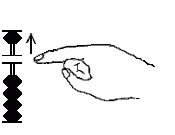Given below are some examples to explain how to solve problems of addition and subtraction.

Simple addition is performed on the abacus by counting the beads for the first number and then counting the beads for the number to be added.

For example, to solve 1 + 2, you would first move up a bead in the lower deck to represent 1, and then move up 2 beads from the lower deck. The beads will then represent the number 3, which is the solution to 1 + 2.

If counting during addition results in a value greater than 10 on any wire, then “carrying” is accomplished by clearing beads from the upper and lower deck of the current wire and moving one bead up from the lower deck on the wire to the left.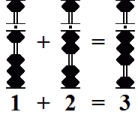To know more, read Complete Guide: How to add two numbers using Abacus?.

Simple Subtraction

Subtraction is done on the abacus by counting out the first number and then clearing beads that represent the second number. For the problem 9 – 6, you would move down one bead in the upper deck and move up four beads in the lower deck to represent the number 9.  Then you would move down 1 bead from the upper deck and move up one bead from the lower deck to subtract 6. The resulting beads represent the number 3, which is the solution to 9 –6.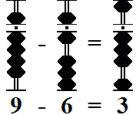To know more, read Complete Guide: How to subtract two numbers using Abacus?.

### Abacus Level 2

Abacus level 1 teaches one about handling simple additions and subtraction while level 2 deals with larger numbers in calculations. When a situation arises where the rods do not contain enough beads to complete addition or subtraction complementary numbers are used. One technique commonly employed is the use of complementary numbers in relation to 5 and 10.

In the case of 5, 2 groups of complementary numbers are used i.e.

4 and 1

3 and 2

In the case of 10, 5 groups of complementary numbers are used i.e.

9 and 1

8 and 2

7 and 3

6 and 4

5 and 5

In addition, the complement is always subtracted:

E.g: 4+8=12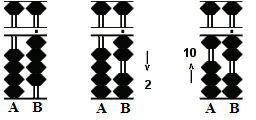Set 4 on rod B, add 8.

Rod B does not have an 8 available, therefore the complementary number is used. As illustrated above the complementary number for 8 is 2

Subtract 2 from 4 on rod B and carry to rod A.

Therefore 4+8=12 becomes 4-2+10=12

### Subtraction using complementary numbers

In subtraction the complement is always added:

E.g: 11-7=4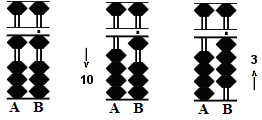Set 11 on rods A B, subtract 7.

As Rod B only has a value of 1, therefore the complementary number is used. As illustrated earlier, the complementary number for 7 is 3.

Subtract 1 from the tens rod on A. Add the complementary number 3 to rod b to give 4.

Therefore 11-7=4 becomes 11-10+3=4

Points to remember:

For addition, first subtract the complement from the rod on the right, and then add a bead to the rod on the left.

For subtraction, first subtract a bead from the rod on the left, and then add the complement to the rod on the right.

## Summary

One might well ask what is the need of learning the abacus when we have such advanced technology these days. The signiﬁcance of Abacus has increased as we have come to rely on modern technology so much that we tend to rely less on our brains for arithmetic calculations or analytical reasoning.

Tools like abacus play a very vital role in developing cognitive skills and stimulate brain development at a very early age. Last but not least it is an excellent tool for removing the fear of numbers in children.

This stimulates brain development at an early age and more importantly is an aid for removing the fear of numbers in children.

Abacus Blogs
Abacus Anatomy & Construction Abacus History
Abacus Level I Abacus Level II
Abacus Counting Abacus Techniques
Abacus Multiplication Abacus Division
Negative Numbers in Abacus Abacus Basics

Cuemath, student-friendly mathematics and coding platform, conducts regular Online Live Classes for academics and skill-development, and their Mental Math App, on both iOS and Android, is a one-stop solution for kids to develop multiple skills.

Check out the fee structure for all grades and book a trial class today!

## What is an abacus?

It is a counting frame used for mathematical calculations.

## Where was the Abacus invented?

The type of Abacus most commonly used today was invented in China around the 2nd century B.C. However, Abacus-like devices are first attested from ancient Mesopotamia around 2700 B.C.!

## How to use an abacus?

Slide all beads to the outer edge of their columns, so no beads are touching the center bar. Use each column of the abacus to represent one digit in the number being calculated, so the right-most column in a zero decimal number is the ones column, the next column to the left is the tens column, and so on.

## What is the difference between Level 1 and Level 2 of learning abacus?

Level 1 deals with handling simple additions and subtraction while level 2 deals with larger numbers in calculations. Use of complementary numbers in calculations.

## What are the advantages of learning how to use an Abacus?

Mathematical skills lay a secure foundation for higher classes.
Abacus education improves the skills of

• Visualization (photographic memory)
• Concentration
• Listening Skills
• Memory, Speed
• Accuracy
• Creativity
• Self Confidence
• Self-Reliance resulting in Whole Brain Development

## External References

Related Articles
Award-winning math curriculum, FREE for a year